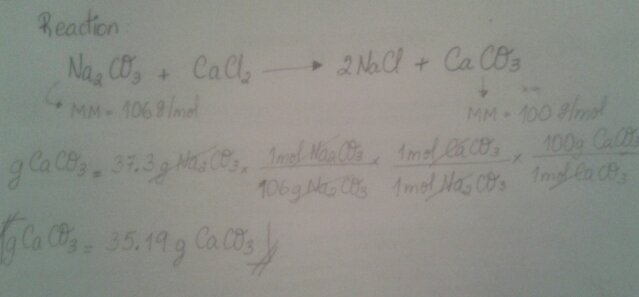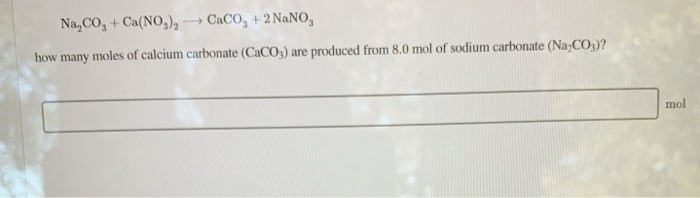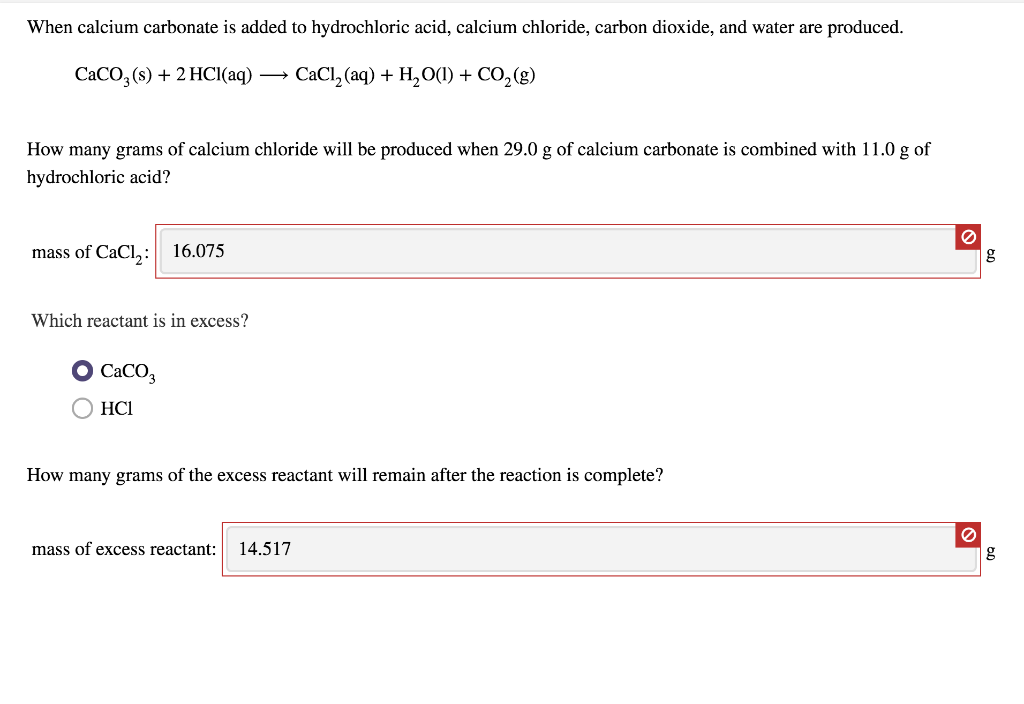Question

# In the following reaction, how many grams of calcium carbonate, CaCO3, will be produced from 37.3...

In the following reaction, how many grams of calcium carbonate, CaCO3, will be produced from 37.3 g of sodium carbonate, Na2CO3?

Supposing the reaction between sodium carbonate and calcium chloride to produce calcium carbonate.#### Earn Coins

Coins can be redeemed for fabulous gifts.

Similar Homework Help Questions
• ### For the reaction Na2CO3+Ca(NO3)2⟶CaCO3+2NaNO3 how many grams of calcium carbonate, CaCO3, are produced from 19.9 g...

For the reaction Na2CO3+Ca(NO3)2⟶CaCO3+2NaNO3 how many grams of calcium carbonate, CaCO3, are produced from 19.9 g of sodium carbonate, Na2CO3?

• ### For the chemical reaction Na2CO3+Ca(NO3)2⟶CaCO3+2NaNO3Na2CO3+Ca(NO3)2⟶CaCO3+2NaNO3 how many moles of calcium carbonate (CaCO3) are produced from 2.02.0...

For the chemical reaction Na2CO3+Ca(NO3)2⟶CaCO3+2NaNO3Na2CO3+Ca(NO3)2⟶CaCO3+2NaNO3 how many moles of calcium carbonate (CaCO3) are produced from 2.02.0 mol of sodium carbonate (Na2CO3)?

• ### For the reaction Na2CO3+Ca(NO3)2⟶CaCO3+2NaNO3 how many grams of calcium nitrate, Ca(NO3)2, are needed to react completely...

For the reaction Na2CO3+Ca(NO3)2⟶CaCO3+2NaNO3 how many grams of calcium nitrate, Ca(NO3)2, are needed to react completely with 55.5 g of sodium carbonate, Na2CO3?

• ### Na,Co, + Ca(NO3)2 + Caco, +2 NaNO, how many moles of calcium carbonate (CaCO3) are produced...Na,Co, + Ca(NO3)2 + Caco, +2 NaNO, how many moles of calcium carbonate (CaCO3) are produced from 8.0 mol of sodium carbonate (Na2CO3)? mol

• ### how many grams of calcium carbonate, CaCO₃, are produced from 76.9 g of sodium carbonate, Na₂CO₃ ?

For the reactionNa₂ CO₃+Ca(NO₃)₂ → CaCO₃+2 NaNO₃how many grams of calcium carbonate, CaCO₃, are produced from 76.9 g of sodium carbonate, Na₂CO₃ ?

• ### When calcium carbonate is added to hydrochloric acid, calcium chloride, carbon dioxide, and water are produced. CaCO3(s...When calcium carbonate is added to hydrochloric acid, calcium chloride, carbon dioxide, and water are produced. CaCO3(s) + 2 HCl(aq) → CaCl2 (aq) + H2O(1) + CO2(8) How many grams of calcium chloride will be produced when 29.0 g of calcium carbonate is combined with 11.0 g of hydrochloric acid? mass of CaCl,: 16.075 g Which reactant is in excess? Caco OHCI How many grams of the excess reactant will remain after the reaction is complete? mass of excess reactant:...

• ### how many moles of calcium carbonate will be produced from 62.0 g of sodium carbonate?

For the chemical reactionNa₂CO₃+Ca(NO₃)₂→CaCO₃+2NaNO₃how many moles of calcium carbonate will be produced from 62.0 g of sodium carbonate?

• ### When calcium carbonate is added to hydrochloric acid, calcium chloride, carbon dioxide, and water are produced....

When calcium carbonate is added to hydrochloric acid, calcium chloride, carbon dioxide, and water are produced. CaCO3(s)+2HCl(aq)⟶CaCl2(aq)+H2O(l)+CO2(g) How many grams of calcium chloride will be produced when 31.0 g of calcium carbonate is combined with 15.0 g of hydrochloric acid? How many grams of the excess reactant will remain after the reaction is complete?

• ### When calcium carbonate is added to hydrochloric acid, calcium chloride, carbon dioxide, and water are produced....

When calcium carbonate is added to hydrochloric acid, calcium chloride, carbon dioxide, and water are produced. CaCO3(s)+2HCl(aq)⟶CaCl2(aq)+H2O(l)+CO2(g) How many grams of calcium chloride will be produced when 28.0 g of calcium carbonate is combined with 13.0 g of hydrochloric acid? mass of CaCl2 : g Which reactant is in excess? HCl CaCO3 CaCO 3 How many grams of the excess reactant will remain after the reaction is complete? mass of excess reactant:

• ### When heated, calcium carbonate decomposes to yield calcium oxide and carbon dioxide gas via the reaction CaCO3 ---> CaO + CO2 How many grams of calcium carbonate are needed to produce 73.0 of carbon dioxide at STP

When heated, calcium carbonate decomposes to yield calcium oxide and carbon dioxide gas via the reaction CaCO3 ---> CaO + CO2 How many grams of calcium carbonate are needed to produce 73.0 of carbon dioxide at STP?